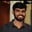Related Tags

recursion
fibonacci
communitycreator
c

# How to find the nth term of the Fibonacci series using recursionBuchiredddypalli Koushik

### Fibonacci series

Let’s first see what the Fibonacci series is:

The Fibonacci series generally starts with 1 and 1 as default, which means that the first and second term is always 1.

The series looks like this:

1,1,2,3,5,8,13...


We can see that the first and the second term is one, and the succeeding term is calculated by the addition of the previous two terms.

So to get the sequence, we need to add the previous two elements. The sequence will be generated in the same way throughout the process.

In this shot, we’ll implement the Fibonacci series using recursion.

Note: Recursion is the process in which the function calls itself. We use recursion here to implement the $n^{th}$ term of the Fibonacci series.

### Example

The 1st term of the Fibonacci series is 1.

• Here n is 1 so if n==1||n==2 we return the value 1.

The 5th term of the Fibonacci series is 5.

• Here, n is 5, so we call fib(n-1)+fib(n-2) recursively to get the value 5.

### Algorithm

• If n is 0, then return 0.

• If n is 1 or 2, we return 1.

• Otherwise, we use fib(n-1)+fib(n-2), which will be called recursively throughout the process.

### Pseudocode

if(n==0 or n==1)

{
return 1
}

return fib(n-1)+fib(n-2);

Recursive tree to calculate Fibonacci of 5
#include<stdio.h>
int fib(int); // Function declaration
int main()
{
int n; // Declaration of variable
scanf("%d",&n);
printf("The %d term in the fibnoci series is: %d ",n,fib(n)); // Calling function
}
int fib(int n) // Function declaration
{
if(n==0)
{
return 0;
}
if(n==1||n==2)
{
return 1;
}
return fib(n-1)+fib(n-2); // Recursive funnction
}

Enter the input below

nth term of Fibonacci Series

### Explanation

First, we read the value of n.

• Line 2: We declare int fib(int).

• Line 7: We call the function fib(n).

• Line 9: We declare the function.

• Line 11–14: If n is 0, we return 0.

• Line 15–18: If n is 1 or 2, we return 1.

• Line 19: If n is something other than 0, 1, or 2 then we call fib(n-1)+fib(n-2) recursively.

RELATED TAGS

recursion
fibonacci
communitycreator
c

CONTRIBUTORBuchiredddypalli Koushik
RELATED COURSES

View all Courses

Keep Exploring

Learn in-demand tech skills in half the time